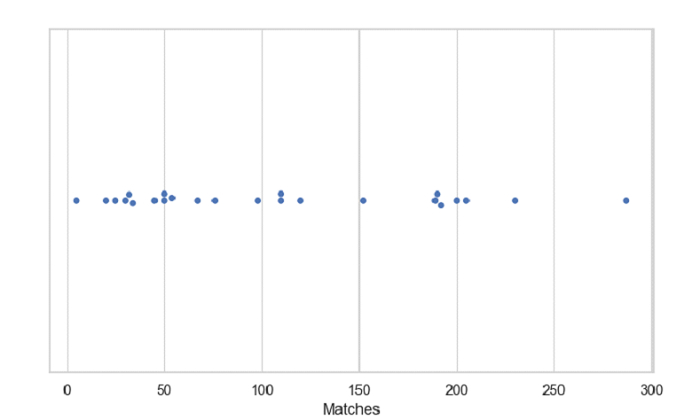# Python - Draw a single horizontal swarm plot with Seaborn

Swarm Plot in Seaborn is used to draw a categorical scatterplot with non-overlapping points. The seaborn.swarmplot() is used for this. Let’s say the following is our dataset in the form of a CSV file − Cricketers2.csv

At first, import the required libraries −

import seaborn as sb
import pandas as pd
import matplotlib.pyplot as plt

Load data from a CSV file into a Pandas DataFrame −

dataFrame = pd.read_csv("C:\Users\amit_\Desktop\Cricketers2.csv")


Plotting swarm plot with the “Matches” column −

sb.swarmplot(x = dataFrame["Matches"])

## Example

Following is the code −

import seaborn as sb
import pandas as pd
import matplotlib.pyplot as plt

# Load data from a CSV file into a Pandas DataFrame

# setting theme
sb.set_theme(style="whitegrid")

# plotting swarm plot with Matches
sb.swarmplot(x = dataFrame["Matches"])

# display
plt.show()

## Output

This will produce the following output −